Next: Exercises Up: Lunar motion Previous: Regression of lunar ascending

# Historical note

It is interesting to note that Clairaut's initial lunar theory, produced in 1747, neglected the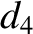contributions to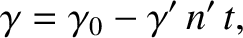and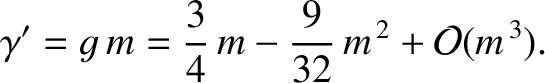--see Equations (11.338) and (11.340), respectively--leading to the prediction that the lunar perigee should precess at the same rate at which the ascending node regresses, and that both the perigee and the node should complete full circuits around the Earth every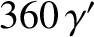years (Taton and Wilson 1995). Of course, this prediction was in serious disagreement with observations, according to which the lunar perigee precesses at about twice the rate that the ascending node regresses. This discrepancy between theory and observations lead Clairaut to briefly doubt the inverse-square nature of Newtonian gravity. Fortunately, Clairaut realized in 1748 that the discrepancy could be resolved by carrying his expansion to higher order in. Indeed, as is clear from Equations (11.338) and (11.340), thecontributions toandgive rise to a significant increase in the precession rate of the lunar perigee relative to the regression rate of the ascending node. In fact, the complete expression for(Delaunay 1867, Hill 1894),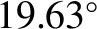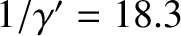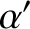(11.341)

takes the form of a power series in. Despite the fact that, for the case of the Moon,takes the relatively small value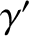, this series only converges very slowly to the value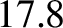(Cook 1988), which corresponds to a precession period (for a full circuit) of the Moon's perigee of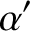years. This estimate lies within 1 percent of the observed precession period [i.e., 8.848 years (Yoder 1995)]. The residual disagreement between theory and observations is largely due to the neglect of small terms involving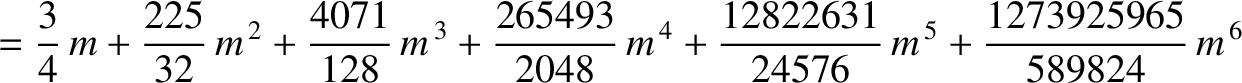,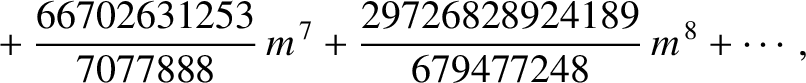,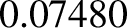, and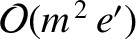in the previous expression (Delaunay 1867). The complete expression for(Delaunay 1867),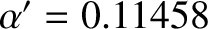(11.342)

also takes the form of a power series in. Fortunately, for the case of the Moon, this series converges relatively quickly to the value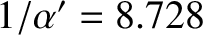(Cook 1988), which corresponds to a regression period (for a full circuit) of the Moon's ascending node of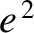years. This estimate lies within half a percent of the observed regression period [i.e., 18.615 years (Yoder 1995)]. Again, the residual disagreement between theory and observations is largely due to the neglect of small terms involving,,, andin the previous expression (Delaunay 1867). The much faster convergence of series (11.342) compared to series (11.341) accounts for the fact that our prediction for the regression period of the lunar ascending node is considerably more accurate than that for the precession period of the perigee.Next: Exercises Up: Lunar motion Previous: Regression of lunar ascending
Richard Fitzpatrick 2016-03-31